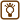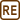## Classical model of spin

We will assume the classical point of view of the electron as a small ball rotating around its axis with radius

$r_\mathrm{c} = \frac{e^\mathrm{2}}{4 \pi \varepsilon_\mathrm{0} mc^\mathrm{2}} \dot = 2.81·10^{\mathrm{-15}} \mathrm{m} \, .$

This is considered to be the so-called classical radius of the electron. This radius can be obtained with assumption of the mass of the electron to be connected with the energy of its electrostatic field by the formula $$E=m{c^\mathrm{2}}$$. We also assume that it spins with angular momentum $$\frac{\hbar}{2}$$. How fast (in m s-1) would the point on the electron’s „equator“ be moving? Does this model make sense?

Note: The real value of electron’s radius was experimentally measured as way smaller than $$r_\mathrm{c}$$ but this makes the situation even worse.

• #### Necessary constants

 $$m$$ = 9.11·10-34 kg electron’s mass $$\varepsilon_\mathrm{0}$$ = 8.85·10-12 F m−1 vacuum permittivity $$\hbar$$ = 1.055·10-34 J s-1 reduced Planck’s constant $$e$$ = 1.6·10-19 C magnitude of the elementary charge $$c$$ = 3·108 m s-1 speed of light in vacuum
• #### Hint 1

Find out how angular momentum is connected with a angular velocity, respectively with tangential speed of the point on the sphere’s equator.

• #### Hint 2

Remind yourself or find out how to determine the moment of inertia $$J$$ for a sphere.

• #### Solution

We will use the relation between the angular momentum $$L$$ and the angular velocity $$\omega$$ $L = J \omega \, ,$ where $$J$$ is the moment of inertia and we can rewrite tangential velocity $$v = r_\mathrm{c} \, \omega$$ as

$v=r_\mathrm{c} \frac{L}{J} \, .$

We will substitute the moment of inertia for rigid sphere $$J = \frac{2}{5}mr_\mathrm{c}^2$$ (see solution of hint 2 above) to the formula for peripheral velocity and simplify

$v=r_\mathrm{c} \frac{\frac{\hbar}{2}}{\frac{2}{5}mr_\mathrm{c}^2}= \frac{5 \hbar}{4mr_\mathrm{c}} \, .$

We will substitute for radius $$r_c$$ to the obtained formula, simplify it and calculate the value of the velocity

$v= \frac{5 \hbar}{4m \frac{e^2}{4 \pi \varepsilon_\mathrm{0} m\mathrm{c}^2}} = \frac{5 \pi \varepsilon_\mathrm{0} \hbar c^2}{e^2} \dot= 5.15·10^{10} \mathrm{m s^{-1}} \, .$

The fastest point on the surface of the electron would be moving with velocity 5.15$$\cdot$$1010 m s-1.

This is approximately hundred times bigger than the speed of light. Since we didn’t consider relativity in our calculations we cannot make any assumption when it comes to deciding if it is possible or not. When applying relativity with the same angular momentum we would get different angular velocity (as with momentum we would obtain two velocities with classical and relativity calculations). Still it gives us a hint that this model doesn’t look realistic.

• #### Note – the derivation of the classical electron’s radius

The classical electron’s radius can be derived with assumption that energy of its electrostatic field corresponds to its rest energy $$E = m {c}^2$$.

We assume the electron to be a small rigid ball with elementary charge $$e$$ equally spread on its surface. We will determine the energy $$E_\mathrm{p}$$ of its electrostatic field with following formula

$E_\mathrm{p} = e \varphi \, ,$

where $$\varphi$$ is electric potential.

Since we know where the charge is we will substitute phi with electric potential on the surface of homogenously charged sphere with radius $$r_\mathrm{c}$$ that we know from the task Field of homogenously charged sphere. From there we know that it corresponds to the potential of point charge in the centre of the sphere. We get the energy of the electron’s electrostatic field

$E_\mathrm{p} = e \frac{e}{4 \pi \varepsilon_\mathrm{0}} \, \frac{1}{r_\mathrm{c}} = \frac{e^2}{4 \pi \varepsilon_\mathrm{0}} \, \frac{1}{r_\mathrm{c}} \, .$

Now we will compare it with rest energy and derive the radius $$r_\mathrm{c}$$

$E_\mathrm{p} = E \, ,$ $\frac{e^2}{4 \pi \varepsilon_\mathrm{0}} \, \frac{1}{r_\mathrm{c}} = m {c}^2 \, ,$ $r_\mathrm{c} = \frac{e^2}{4 \pi \varepsilon_0 m{c}^2} \, .$

We obtained the relation given in the task assignment.×Original source: GRIFFITHS, David J. Introduction to Quantum Mechanics. 2nd ed. Upper Saddle River: Pearson Prentice Hall, 2005